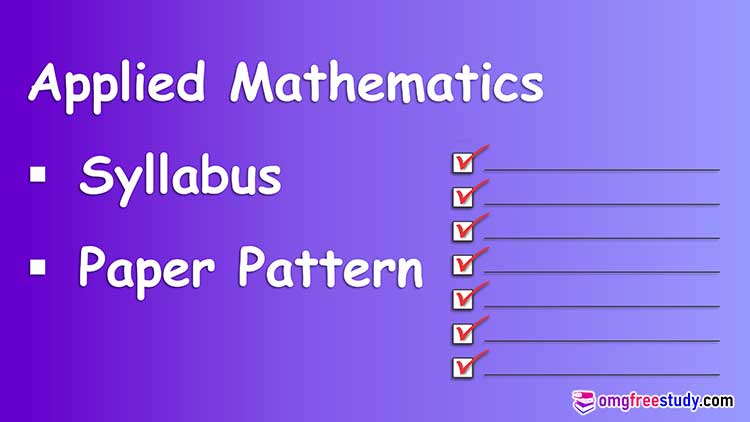# Applied Mathematics Syllabus & Paper Pattern

On this page we are trying to present to you Applied Mathematics Syllabus and Paper Pattern with marking scheme. Which is helpful for Polytechnic or Diploma Engineering students, who’s presently study in first year second semester ‘I’ Scheme under MSBTE (All Branch).## Tutorials

Solve problems based on finding value of the function at different points, find derivatives of implicit function and parametric function, find derivative of logarithmic and exponential functions.

Problems based on finding equation of tangent and normal, finding maxima and minima of function and radius of curvature at a given point.

Methods of integration, substitution, partial fractions and integration by parts.

Properties of definite integration, finding area under curve, area between two curves and volume of revolutions.

Formation, order and degree of differential equations. Concept of linear differential equation to related engineering problem. All above syllabus are common for all engineering branches and last topic different for all groups.

## Applied Mathematics Syllabus

2 ND Semester Diploma Engineering for All Branches.

Units and subunit of applied mathematics are listed below (Total Marks 70).

1) Differential calculus (24 Mark)

a) Functions and Limits

b) Derivatives

c) Applications of derivative

2) Integral calculus (16 Mark)

3) Applications of Definite Integration (8 Mark)

a) Definite Integration

b) Applications of Definite integration

4) Differential Equations (8 Mark)

After that next unit of applied mathematics are different for different groups of engineering branch.

Electrical Group (14 Mark)

5) Complex numbers and Laplace transform

Mechanical Group (14 Mark)

5) Probability distribution.

Civil Group (14 Mark)

5) Numerical integration

## Applied Mathematics Paper Pattern

All above topics of applied mathematics are distributed in different question number with different marking, which is listed below.

Que. 1. Attempt any Five (10 Mark)

Que. 2. Attempt any Three (12 Mark)

Que. 3. Attempt any Three (12 Mark)

Que. 4. Attempt any Three (12 Mark)

Que. 5. Attempt any Two (12 Mark)

Que. 6. Attempt any Two (12 Mark)

Download PDF of MSBTE Syllabus of Second Semester For All branch. Applied Mathematics.

Watch Video Lecture on Syllabus and Paper Exam Pattern on

Scroll to Top
Scroll to Top